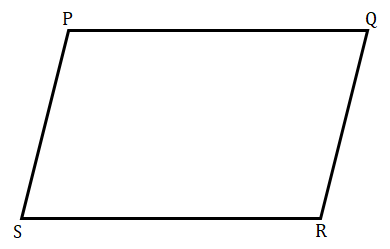# Two adjacent angles of a parallelogram have equal measure. Find the measure of each of the angles of the parallelogram.

Given: Two adjacent angles of a parallelogram have equal measure.

To find: Here we have to find the measure of each of the angles of the parallelogram.

Solution:

Let PQRS be a parallelogram;Two adjacent angles of a parallelogram have equal measure. So,

∠P = ∠Q

Now,

Sum of adjacent angles of a parallelogram = 180°

∠P $+$ ∠Q = 180°

2∠P = 180°

P = 90°

Also,

90° $+$ ∠Q = 180°

∠Q = 180° $-$ 90°

∠Q = 90°

So,

∠P = ∠R = 90°

∠Q = ∠S = 90°

So, all the angles of parallelogram are equal to 90°.

Updated on: 10-Oct-2022

25 Views